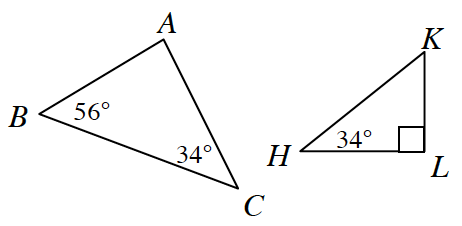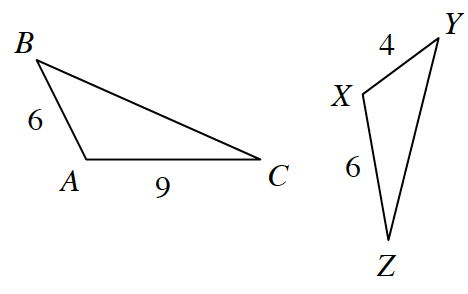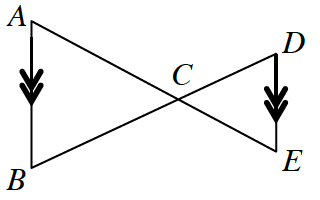### Home > GC > Chapter 10 > Lesson 10.1.3 > Problem10-32

10-32.

For each pair of triangles below, decide if the triangles are similar or not and explain how you know. If the triangles are similar, complete the similarity statement $ΔABC \sim Δ\text{___}$.

1.By $AA\sim$, $ΔABC \sim ΔLKH$

1.There is not enough information to determine whether or not the two triangles are similar.

1.$∠A\text{ and }∠E$ are alternate interior angles, as are $∠B\text{ and }∠D$.